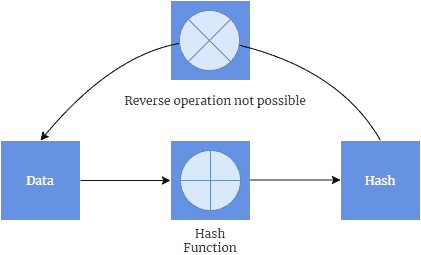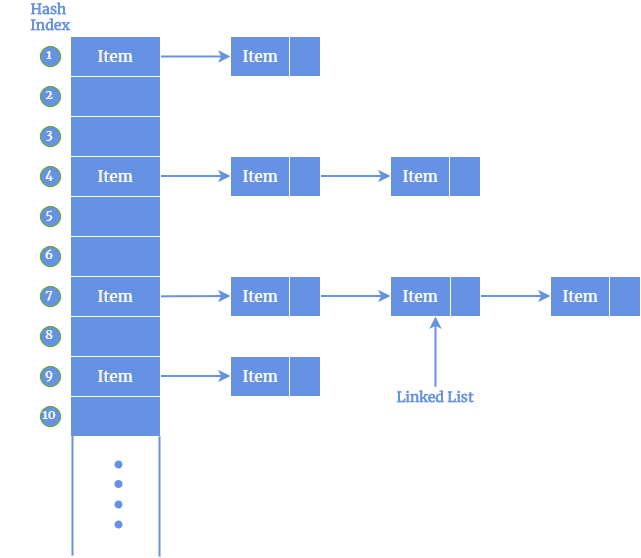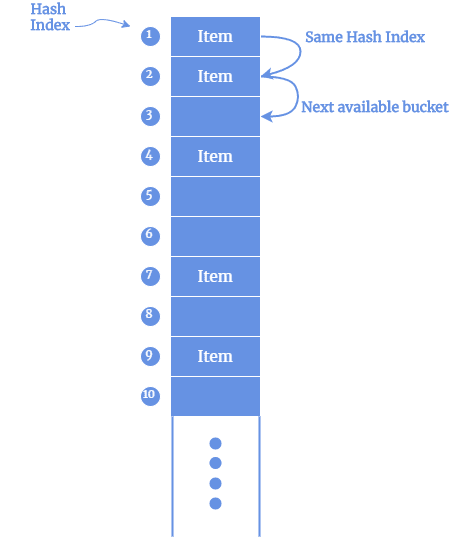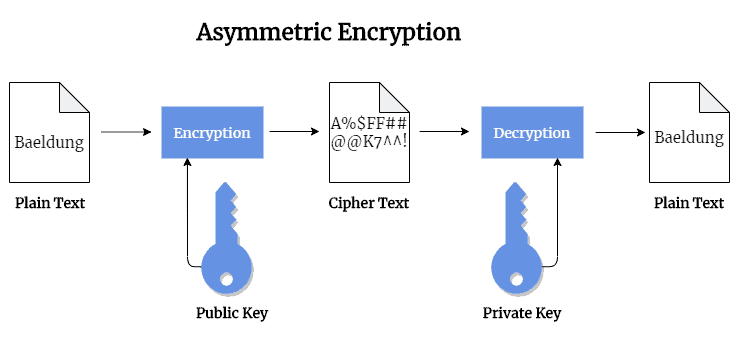## 1. Introduction

Hashing and encryption are the two most important and fundamental operations of a computer system. Both of these techniques change the raw data into a different format. Hashing on an input text provides a hash value, whereas encryption transforms the data into ciphertext.

Although both of these techniques convert the data into a separate format, there are subtle differences in their conversion mechanism and area of usage.

In this tutorial, we’ll discuss these techniques and their differences.

## 2. What Is Hashing?

Hashing is the process of mapping any arbitrary size data into a fixed-length value using a hash function. This fixed-length value is known as a hash value, hash code, digest, checksum, or simply hash. Computer systems use hashing in two major areas:

• To calculate the integrity of a file or message during transfer over the network. For instance, user A can send a file to user B and provide the hash value along with the original message. User B can calculate the hash value of the received file. A match of both hash values assures user B that the file integrity is intact
• Another use of hashing is in a hash table. A hash table is a data structure that stores data with the associated hash value as the table index and the original data as a value

### 2.1. What Is a Hash Function?

A hash function is an underlying algorithm that computes the hash value of the supplied data. One of the interesting features of a hash function is that it is a one-way algorithm. We can compute the hash value from the give data, but the reverse operation is not possible. Thus, it is not possible to take a hash value and reconstruct the message from it:### 2.2. Requirements for Good Hash Function?

Before exploring the available hash functions, let’s explore the characteristics of a good hash function:

• Deterministic: A hash function should be deterministic in nature. This means that for a given input the hash function must produce the same hash value
• Input Usage: A hash function also should use most of the input data. This ensures that it can produce a distinct hash value for similar inputs
• Uniform Distribution: Hash tables use the hash value as the table index to store data. Thus, a good hash function should ensure that the hash value distributes uniformly across the table
• Distinct Has: A hash function should produce different hash values for similar strings

### 2.3. Hash Function Examples

There are several such algorithms available to compute the hash value such as the division method, identity hash function, multiplication method.

Let’s explore two popular hashing algorithms: Division Hashing and Identity Hash Function.

In the Division Hashing algorithm, we map the key into one of the slots of the hash table by taking the remainder of the key divided by table size. In mathematical notations, this is represented as. The value of m is a primer number so that it can generate unique hash values. The table size is usually chosen as a power of two and provides a distribution fromto.

Although this is a simple algorithm, it has two major drawbacks.

• The modern computer system uses multiplication to implement division operation. Thus, the division is a relatively slower operation
• For certain keyset, this algorithm produces the same hash value. For instance, consider the keys 134000. 156000, and 145000 with mod 1000. All 3 key produces the same hash value and thus maps to the same slot in the table

In the identity hash function, the data itself is the hash value. This algorithm is suitable for small datasets. The meaning of small in this context depends on the size of the data that needs to be used as the hashed value. For example, In Java, a hashcode is a 32-bit integer. Thus, 32-bit Integer and 32-bit Float objects can use the value directly. However, 64-bit Long and 64-bit Double objects can’t use this algorithm.

There are two benefits of this algorithm:

• The cost of computing the hash value is effectively zero as the value itself is the hash value and there is no computation
• This is a perfect hash function as there are no collisions in the hash value

### 2.4. Collisions

While computing hash value using hash functions, we also need to deal with collisions. A collision occurs when the hash function returns the same hash value for two distinct input data. A good hash function ensures there are fewer collisions. There are several techniques to address collisions.

• Open Hashing: In this technique, data is not directly stored in the hash key index in the hash table. A separate data structure such as a linked list stores the data. Thus, if two separate input data maps to the same hash index in the hash table, a linked list stores the input values in a linear fashion:• Closed Hashing: In this alternate technique, no additional data structure is used to manage collisions. In the event of collisions, we try to find the alternate buckets in the same hash table until an empty cell is available:## 3. Encryption

Data encryption is the process that translates the data from its original form to another form. The original form of data is known as plaintext and the encrypted form of data is known as ciphertext. The ciphertext is decrypted by a secret key.

### 3.1. Need for Data Encryption

The main purpose of encrypting data is to protect data confidentiality while it is stored on computer systems or transmitted to other computers over the network. Modern data encryption algorithms ensure data confidentiality and provide key security features including authentication, integrity, and non-repudiation.

The authentication feature allows the verification of a message’s origin. The integrity feature ensures that a message’s contents have not changed since it was sent. Additionally, non-repudiation guarantees that a message sender cannot deny sending the message.

### 3.2. How Data Encryption Works?

In the data encryption process, relevant data is encrypted with an encryption algorithm and an encryption key. This process results in the ciphertext and can only be viewed in its original form if it is decrypted with the correct key. Based on the key type, there are two main types of encryptions – symmetric encryption and asymmetric encryption.

### 3.3. Symmetric Encryption

Symmetric-key encryption uses the same secret key for encrypting and decrypting the data. The major benefit of this type is that it is much faster than the asymmetric encryption type. However, the demerit is that the sender needs to exchange the encryption key with the recipient so that receiver can decrypt it.

To overcome the additional overhead of securely exchange the secret key, organizations have adapted to use an asymmetric algorithm to exchange the secret key after using a symmetric algorithm to encrypt data.

### 3.4. Asymmetric Encryption:

This encryption type is also referred to as public-key cryptography. This is because two different keys one public and one private key are used in this encryption process. The public key, as it is named, may be shared with everyone, but the private key must be protected:The Rivest-Sharmir-Adleman (RSA) algorithm is a popular public-key encryption that is extensively used to secure sensitive data. The RSA algorithm’s popularity is due to the fact that both the public and private keys can encrypt a message to assure the confidentiality, integrity, authenticity, and non-repudiability of transmitted data.

### 3.5. Challenges of Encryption

Although the encryption algorithms offer to protect the data, it is still often the victim of several attacks. These attacks compromise the underlying promises of encryption. The major type of attack on encryption today is the brute force or trying random keys until the right one is found.

Another mode of popular attack is side-channel. This attack types target the cipher implementation, rather than the actual cipher itself. These attacks tend to succeed if there is an error in system design or execution.

## 4. Conclusion

In this article, we discussed two major features of data security in computer systems – hashing and encryption.

In the beginning, we talked about hashing that computes a unique hash value of the supplied data.

Later, we discussed encryption that converts the data to a ciphertext and can be restored using a secret key.